﻿ VIC Natural logarithm. Natural exponential.

VI.C. The Natural Logarithm and Exponential Functions

In the previous sections we have considered independently the functions that solved two differential equations. The "natural logarithm" function, ln , was the solution to the differential equation L'(t) = 1/t with L(1) = 0 , while the "natural exponential" function, exp or e, was the solution to the differential equation P'(t) = P(t) with P(0) = 1. You probably have seen these two functions before in your pre-calculus course work where they were closely related as the next theorem shows.

Theorem VI.C.1: (a) For all t > 0, exp(ln(t)) = t.  (b) For all x, ln(exp(x)) = x.

Proof: (a) Let F(t) = exp(ln(t))/t for t > 0.

Then using the quotient and the chain rules we see that

F'(t) = [t .exp(ln(t)) .1/t - exp(ln(t)) .1]/t 2 = 0 for all t > 0.

Thus F(t) is a constant and since F(1)= exp(ln(1))/1 = exp(0)/1 = 1 we have

exp(ln(t))/t = 1 or exp(ln(t)) = t for all t > 0.

(b) Let F(x) = ln(exp(x)) - x for all x .
Then using the chain rule we see that F'(x)= 1/exp(x) . exp(x)-1= 0 for all x .
Thus F(x) is a constant. Since F(0) = ln(exp(0)) - 0= ln(1)= 0 we have ln(exp(x)) - x = 0 for all x or ln(exp(x)) = x for all x .EOP

#### Sorry, this page requires a Java-compatible web browser.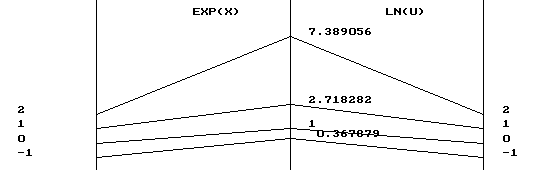We can visualize the relationship described in the theorem in several ways. Using transformation figures as in Figure VI.C.i , we see that the arrows used to visualize the ln function as a transformation reverse the arrows used to visualize the exp function and vice versa.

In functional terms, the composition of ln with exp is the identity function, as is also the composition of exp with ln, i.e.,

ln ° exp = id = exp ° ln,

when the domain is suitably chosen. For both these reasons we say that the natural logarithm and natural exponential function are inversely related as functions.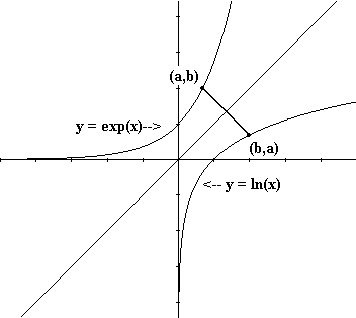Another graphical way to view this inverse relation is to note that if b = exp(a) then ln(b) = ln(exp(a)) = a . Thus if (a,b) is a point on the graph of the natural exponential function, then (b,a) is a point on the graph of the natural logarithm function. Similarly if a = ln(b) , b > 0 then exp(a) = exp(ln(b)) = b which shows that if (b,a) is a point on the graph of the natural logarithm function then (a,b) is a point on the graph of the natural exponential function. See Figure VI.C.ii. We summarize this by saying exp(a) = b if and only if b = ln(a) , or using the notation e a = exp(a),

e a = b if and only if b = ln(a).

This last statement justifies calling the natural logarithm "the logarithm with the base e" and writing in more conventional terms ln(a) = log e(a). For example, e 0 = 1 so, ln(1) = 0 and e1=e so ln(e) = 1. In general ln(e a) = a , which merely restates part (b) of the theorem.

Applications: The inverse relation of these two functions is most convenient for finding ways to express the solutions to differential equations that are in exponential form as the following examples illustrate.

Example VI.C.1: Suppose f(x) = A e kx . Find A and k when f(0) = 20 and f(1) = 40. Find t so that f(t) = 100.

Solution: First we merely substitute the given values into the formula for f: 20 = f(0) = Ae k(0) = A so A=20, while 40=f(1)=A e k(1) =20 e k. So 2 = e k.

Using the inverse relation between ln and exp in this equation means precisely that k = ln(2). Thus f(x) = 20 e (ln(2) x).

To find when f(t) = 100 we need to solve 100 = 20 e (ln(2) t), or 5 = e (ln(2) t). Using the inverse properties once again, we have ln(5) = t ln(2) so

t = ln(5) / ln(2) » 2.32.

Example VI.C.2: Suppose f(x) = Ae kx and f(3) = 2f(0). If f(1) = 40, find f(5).

Solution: f(0) = A so we have been given 2 A = A e k3 in the statement f(3) = 2f(0). Thus 2 = e 3k , so by the inverse relation of ln and exp we have that 3k = ln(2) or k = (1/3) ln(2).

The second equation f(1) = 40 becomes 40 = Ae k. At this point we can solve for A theoretically by dividing both sides of the last equation by e k, giving A = 40 / e k. We continue with this form for A to find f(5) = A e k5 = (40 / e k) e 5k = 40 e 4k = 40 e 4/3 ln(2).

Recognizing this result is not the easiest number to compute, let's notice that

e 4/3 ln(2)  = (eln(2))4/3 = 2 4/3
because of the properties of exponents and the inverse relation of ln and exp.

So f(5) = 40 (2) 4/3.

The connection of the natural logarithm to other core exponential and logarithmic functions.
The natural logarithm function can be defined as a definite integral: `ln(x) = int _1^x  1/t dt` . Using this definition, the natural logarithm can provide the mathematical foundation for all other logarithmic and exponential functions.  The following chart demonstrates the major connections of these functions in one "Big Picture."
 Def'n.:  `ln(x) = int_1^x  1/t dt`   [ x>0] Def'n.:  exp (x) = y `-=` ln(y) = x exp(1) `-=` e [so ln(e) = 1]. exp(x) `-=` ex Def'n.: For b >0,  bx`-=`e xln(b). Note: ln(bx) = x ln(b) Def'n.: For b >0,  log b (x) = y `-=` x= by ln(1) = 0  ln(x) > 0 for x >1  ln(x) < 0 for 0< x <1 exp(0) = e0=1  ex> 1 for x > 0  0< ex < 1 for x < 0 b0 = 1  For b > 1:  b x  > 1  for x > 0  0< bx < 1 for x < 0  For 0< b < 1:  b x  > 1  for x < 0  0< bx < 1 for x > 0 log b (1) = 0  For b > 1:  log b (x)>0 for x >1  log b (x)<0 for 0< x <1  For 0< b < 1:  log b (x)<0 for x >1  log b (x)>0 for 0< x <1 ln(A*B) = ln(A) + ln(B) eAeB =eA+B bAbB = bA+B log b (A*B) = log b (A) + log b (B) ln(A/B) = ln(A) - ln(B) eA / eB = eA-B bA /bB = bA-B log b (A/B) = log b (A ) - log b (B) ln(Ap/q) =p/q ln(A) (ex) p/q = e(p/q)*x (bx) p/q = b(p/q)*x log b (Ap/q )= p/q log b (A) ln'(x) = D ln(x) = 1/x  [So ln is continuous and increasing for x >0] exp'(x) = D(ex) = ex D(bx) = ln(b) bx log b'(x) = D log b (x) = 1/( x ln(b)) `int 1/u du = ln|u| + C` `int e^u du = e^u  + C` ` int b^u du = b^u / ln(b) + C` Not relevant! As `x -> oo , ln (x) -> oo`. As `x -> oo,  e^x -> oo`. b >0: As `x -> oo,  b^x -> oo` b <0:As `x -> oo,  b^x -> 0` For b > 1:As `x -> oo, log_b (x) -> oo`.  For 0< b < 1:As `x -> oo, log_b (x) -> - oo`. As `x -> 0^+ , ln(x) -> - oo` As `x -> - oo , e^x -> 0` b>0: As `x -> - oo , b^x -> 0` b< 0:As `x ->-  oo,  b^x -> oo` For b > 1:As `x -> 0^+, log_b (x) -> - oo`. For 0< b < 1: As `x -> 0^+, log_b (x) -> oo`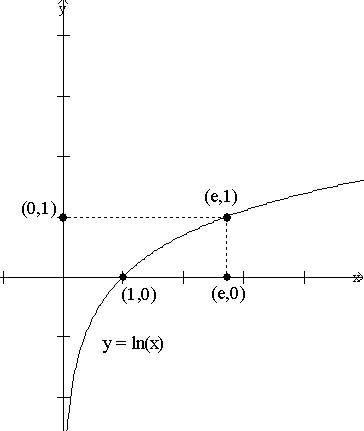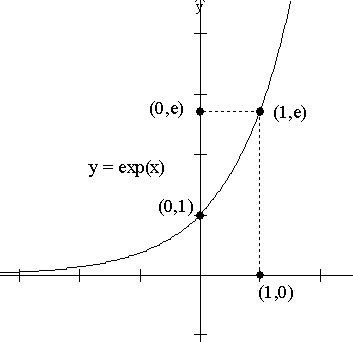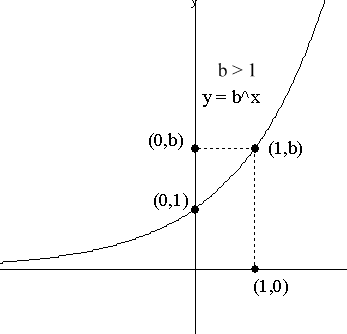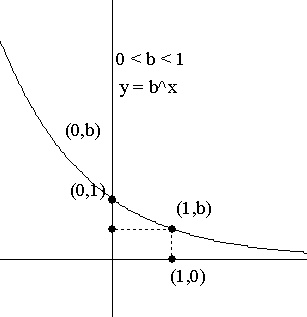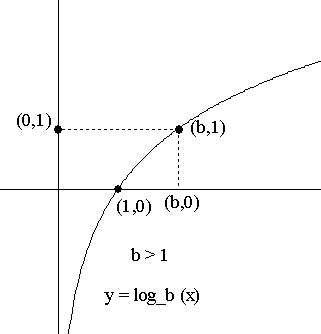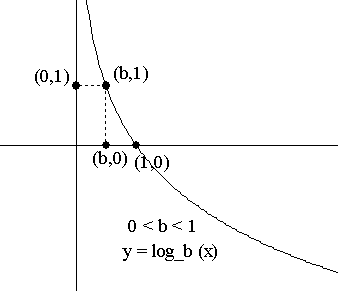Exercises VI.C

Find the first derivative for the functions in problems 1-4 using logarithmic differentiation:

1. f(x) = 7 x
2. f(x) = 3 sin(x)
3. y = sin(x) 5 x
4. `g(t) = t^pi`
5. The numbers `e^pi` and `pi^e` have been of interest to mathematicians for many years. Consider the function  f(x) = e x - x e.

6. Use calculus to determine the local extreme values of  f and when f is increasing or decreasing. With this information determine whether `f(pi)` is positive or negative.
Which is larger, `e^pi` or `pi^e`?

Find the first derivative for the functions in problems 6-9.

7. f(x) = log 5(x)
8. f(x) = log 3(sin(x))
9. y = sin(x) log 5(x)
10. g(t) = log t(2) for t > 1.
11. Find `int 5^x dx`.
12. Find `int 5^{sin(x)}cos(x) dx`.
13. Find `int_e^{e^2}  (ln(x))/x dx`.
14. Find `int_e^{e^2} 5/(ln(x)x) dx`.